# 分数

### 切开披萨，就得到分数：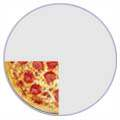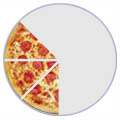1/2 1/4 3/8 （一半） （四分之一） （八分之三）

## 等值分数

 4/8 = 2/4 = 1/2 （八分之四） （四分之二） （一半）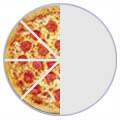=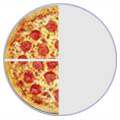=分子 分母

## 分数加法

 1/4 + 1/4 = 2/4 = 1/2 （四分之一） （四分之一） （四分之二） （一半）+==5/8 + 1/8 = 6/8 = 3/4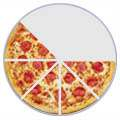+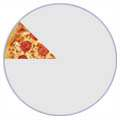=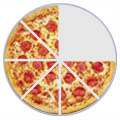=## 加不同分母的分数

 3/8 + 1/4 = ？+=3/8 + 2/8 = 5/8+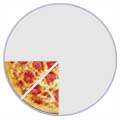=